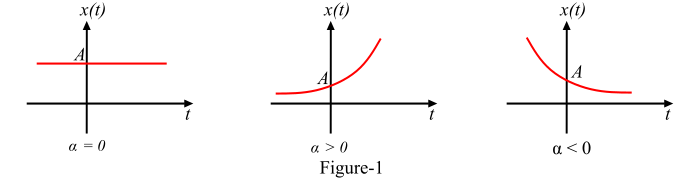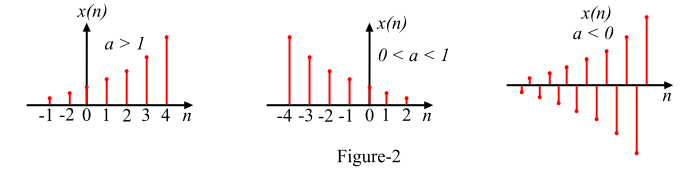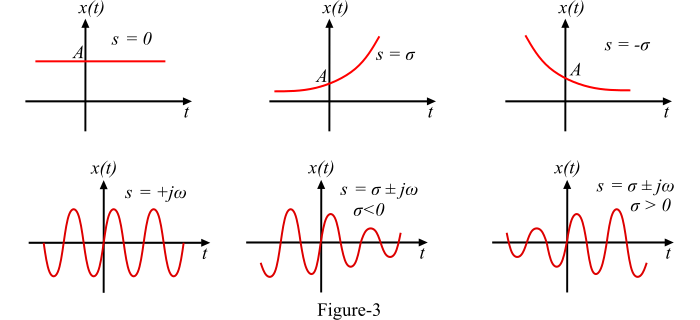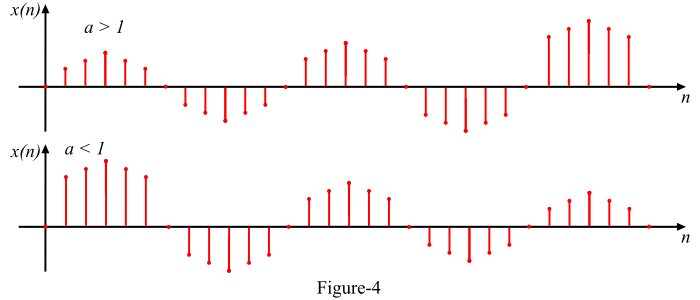# Signals and Systems: Real and Complex Exponential Signals

## Real Exponential Signals

An exponential signal or exponential function is a function that literally represents an exponentially increasing or decreasing series.

## Continuous-Time Real Exponential Signal

A real exponential signal which is defined for every instant of time is called continuous time real exponential signal. A continuous time real exponential signal is defined as follows −

𝑥(𝑡) = 𝐴𝑒𝛼𝑡

Where, A and 𝛼 both are real. Here the parameter A is the amplitude of the exponential signal measured at t = 0 and the parameter 𝛼 can be either positive or negative.

Depending upon the value of 𝛼, we obtain different exponential signals as −

• When 𝛼 = 0, the exponential signal x(t) is a signal of constant magnitude for all times.

• When 𝛼 > 0, i.e., 𝛼 is positive, then the exponential signal x(t) is a growing exponential signal.

• When 𝛼 < 0, i.e., 𝛼 is negative, then the signal x(t) is a decaying exponential signal.

The waveforms of these three signals are shown in Figure-1.## Discrete-Time Real Exponential Signal

A real exponential signal which is define at discrete instants of time is called a discrete-time real exponential signal or sequence. A discrete-time real exponential sequence is defined as −

𝑥(𝑛) = 𝑎𝑛   for all 𝑛

Depending upon the value of a the discrete time real exponential signal may be of following type −

• When a < 1, the exponential sequence x(n) grows exponentially.

• When 0 < a < 1, the exponential signal x(n) decays exponentially.

• When a < 0, the exponential sequence x(n) takes alternating signs.

These three signals are graphically represented in Figure-2.## Complex Exponential Signals

An exponential signal whose samples are complex numbers (i.e., with real and imaginary parts) is known as a complex exponential signal.

## Continuous-Time Complex Exponential Signal

A continuous time complex exponential signal is the one that is defined for every instant of time. The continuous time complex signal is defined as −

𝑥(𝑡) = 𝐴𝑒𝑠𝑡

Where,

• A is the amplitude of the signal.

• s is a complex variable.

The complex variable s is defined as,

𝑠 = 𝜎 + 𝑗𝜔

Therefore, the continuous time complex function can also be written as

𝑥(𝑡) = 𝐴𝑒(𝜎+𝑗𝜔)𝑡 = 𝐴𝑒𝜎𝑡𝑒𝑗𝜔𝑡

⟹ 𝑥(𝑡) = 𝐴𝑒𝜎𝑡(cos 𝜔𝑡 + 𝑗 sin 𝜔𝑡)

Depending upon the values of 𝜎 and 𝜔, we obtain different waveforms as shown in Figure-3.## Discrete-Time Complex Exponential Sequence

A complex exponential signal which is defined at discrete instants of time is known as discrete-time complex exponential sequence. Mathematically, the discrete-time complex exponential sequence is defined as,

$$\mathrm{x(n)=a^{n}e^{j(\omega _{0}n+\varphi )}=a^{n}\cos (\omega _{0}n+\varphi)+ja^{n}\sin (\omega _{0}n+\varphi)}$$

Depending on the magnitude of a, we obtained different types of discrete-time complex exponential signals as,

• For |𝑎| = 1, both the real and imaginary parts of complex exponential sequence are sinusoidal.

• For |𝑎| > 1, the amplitude of the sinusoidal sequence increases exponentially.

• For |𝑎| < 1, the amplitude of the sinusoidal sequence decays exponentially.

The graphical representation of these signals is shown in Figure-4.